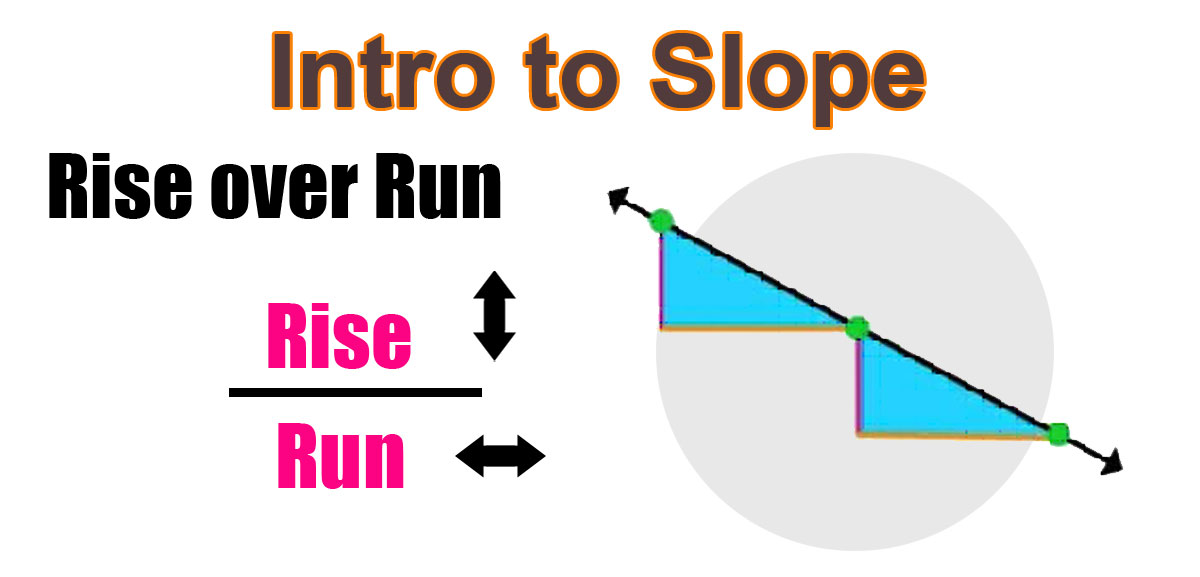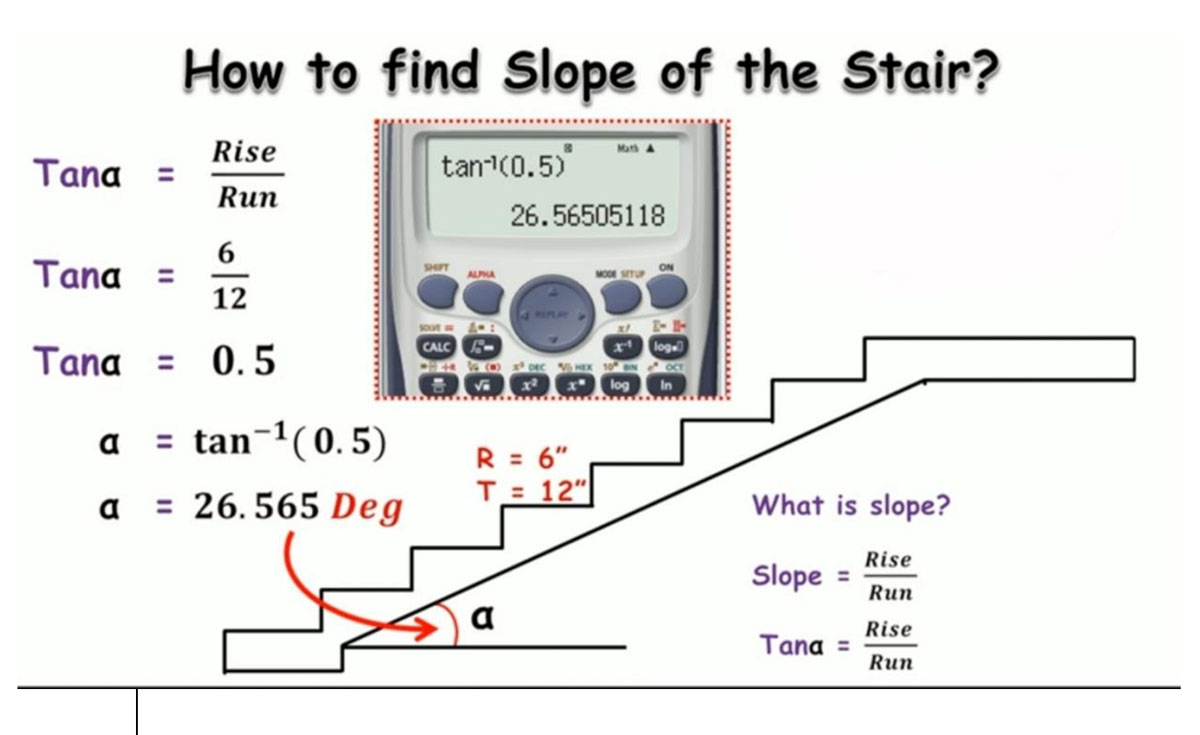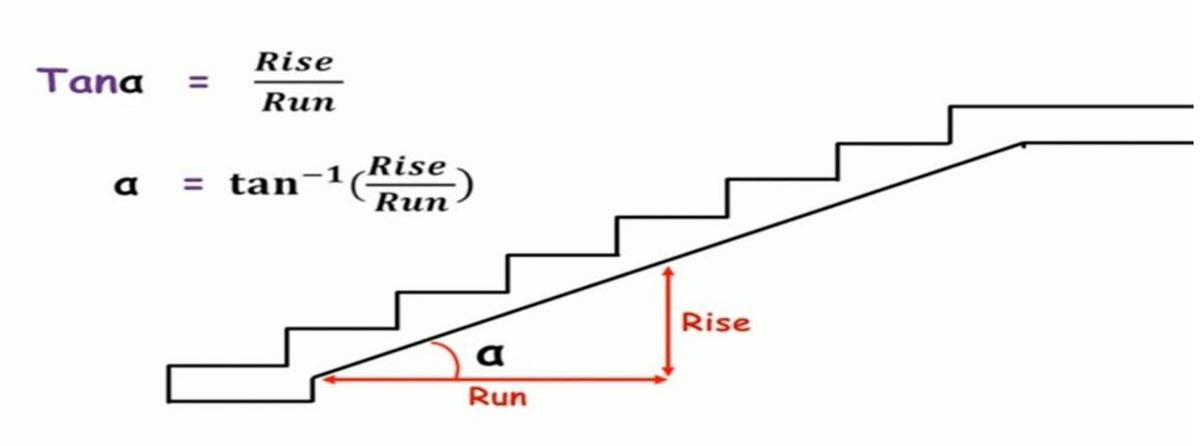# HOW TO CALCULATE THE SLOPE OF A STAIR ?

Staircase is an important component of a building providing access to different floors and roof of the building. It consists of a flight of steps (stairs) and one or more intermediate landing slabs between the floor levels. Different types of staircases can be made by arranging stairs and landing slabs.

First of all we need to understand what does a slope mean? Actually , the slope over here means how much an angle does a stair make with the horizontal reference line. This is just a simple definition of the slope of the stair. The formula to find out the slope is the ratio between the rise and the run.So , now the question is how can we determine same angle which the stair is making with the horizontal reference line?

We will explain this with an example. Let's say we know the rise an run value;

rise is 6" and run is 12

Therefore when we use the formula above , our answer will be tan a = 0.5"{tan a= 6/12} .

Further we calculate the value of tan a in our scientific calculator, we get the value as 26.56505118 degree

{ a= tan -1 (0.5")}.## Now what if the rise and the run of the stairs are unknown?

In this case we have to take few measurements .If you measure horizontal distance from the edge of the stair and the other from the perpendicular distance, we can get the rise and run value by using a measuring tape.Now this is the right angle triangle, so using rise over run ratio, we can find an angle of the stairs.